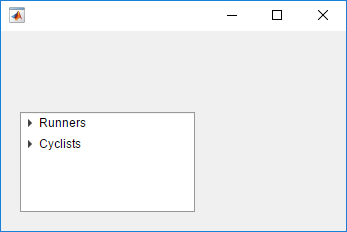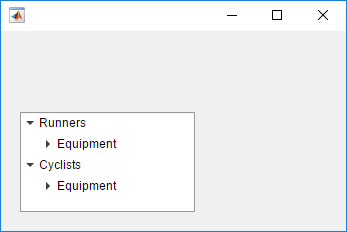Main Content

# expand

Expand tree node

## Syntax

``expand(parent)``
``expand(parent,'all')``

## Description

````expand(parent)` expands the nodes of a tree or tree node. If `parent` is a `Tree` object, then the top-level nodes display in an expanded state. If `parent` is a `TreeNode` object, then that node displays in an expanded state.```

example

````expand(parent,'all')` expands all nodes of a tree or tree node.```

## Examples

collapse all

Create a tree that has three levels of nodes. By default, the nodes display in a collapsed state.

```fig = uifigure; tree = uitree(fig,'Position',[20 20 175 100]); % First level nodes category1 = uitreenode(tree,'Text','Runners'); category2 = uitreenode(tree,'Text','Cyclists'); % Second level nodes equip1 = uitreenode(category1,'Text','Equipment'); equip2 = uitreenode(category2,'Text','Equipment'); % Third level nodes shoes = uitreenode(equip1,'Text','Running Shoes'); bike = uitreenode(equip2,'Text','Bicycle'); helmet = uitreenode(equip2,'Text','Helmet'); ```Expand the top-level nodes.

`expand(tree)`Expand all the nodes in the tree.

`expand(tree,'all')`## Input Arguments

collapse all

Parent tree or node, specified as a `Tree` object or `TreeNode` object.

## See Also

Introduced in R2017b

Download ebook